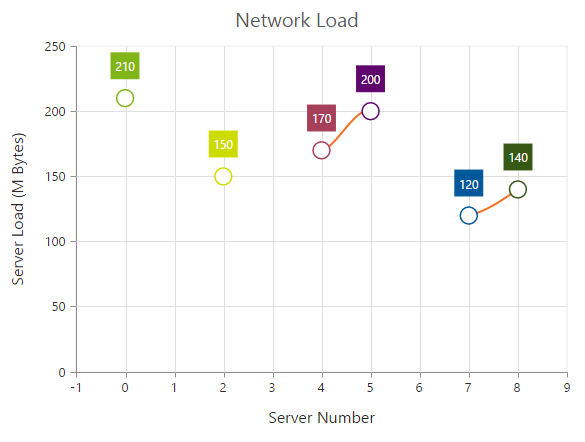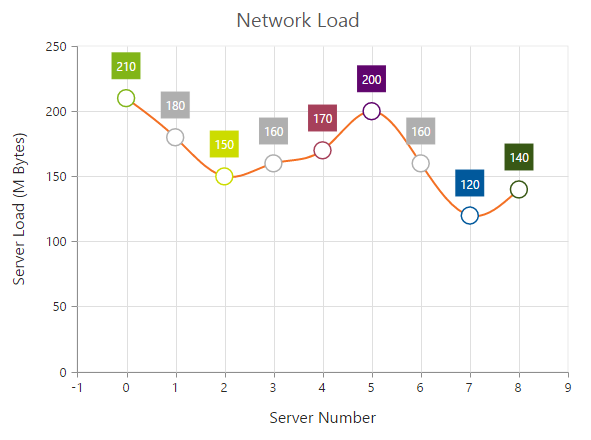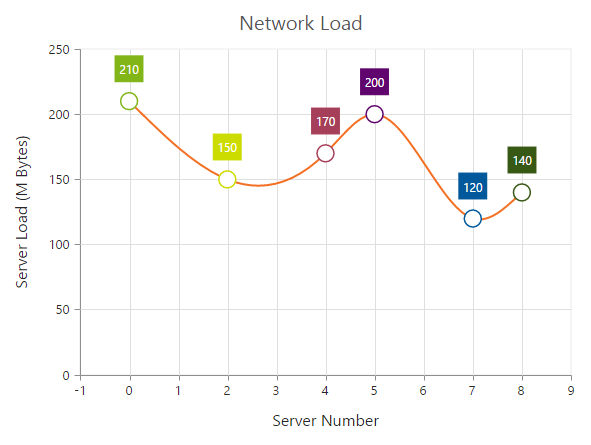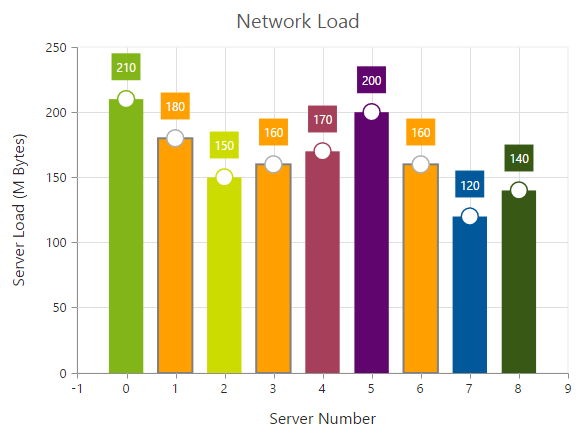# Empty Points

22 Jan 20183 minutes to read

The data points, which uses the null or undefined as value are considered as empty points. Empty data points are ignored and not plotted in the chart. When the data is provided by using the Points property, set the IsEmpty to true to specify the particular point is an empty point.

• CSHTML
• ``````<ej-chart id="chartContainer">
// ...
<e-chart-series>
<e-series>
<e-points>
<e-point x="0" y="210"></e-point><e-point x="1" y="0"></e-point>
<e-point x="2" y="150"></e-point><e-point x="3" y="180" is-empty="true"></e-point>
<e-point x="4" y="170"></e-point><e-point x="5" y="200"></e-point>
<e-point x="6" y="140" is-empty="true"></e-point><e-point x="7" y="120"></e-point>
<e-point x="8" y="140"></e-point>
</e-points>
<e-empty-point-settings visible="true"></e-empty-point-settings>
</e-series>
</e-chart-series>
// ...
</ej-chart>``````## EmptyPointSettings

You can customize the visibility of empty points and change its DisplayMode to Gap, Zero, and Average by using EmptyPointSettings option.

• CSHTML
• ``````<ej-chart id="chartContainer">
// ...
<e-chart-series>
<e-series>
<e-points>
<e-point x="0" y="210"></e-point><e-point x="1" y="0"></e-point>
<e-point x="2" y="150"></e-point><e-point x="3" y="180" is-empty="true"></e-point>
<e-point x="4" y="170"></e-point><e-point x="5" y="200"></e-point>
<e-point x="6" y="140" is-empty="true"></e-point><e-point x="7" y="120"></e-point>
<e-point x="8" y="140"></e-point>
</e-points>
<e-empty-point-settings visible="true" display-mode="Average"></e-empty-point-settings>
</e-series>
</e-chart-series>
// ...
</ej-chart>``````If the Visible property of EmptyPointSettings is false, then the empty points will be dropped and chart will be rendered without empty points.## Customizing styles

Empty points color and border can be customized by using Style property of EmptyPointSettings.

• CSHTML
• ``````<ej-chart id="chartContainer">
// ...
<e-chart-series>
<e-series>
<e-empty-point-settings visible="true">
<e-Style color="pink"><e-border color="gray" width="2"></e-border></e-Style>
</e-empty-point-settings>
</e-series>
</e-chart-series>
// ...
</ej-chart>``````